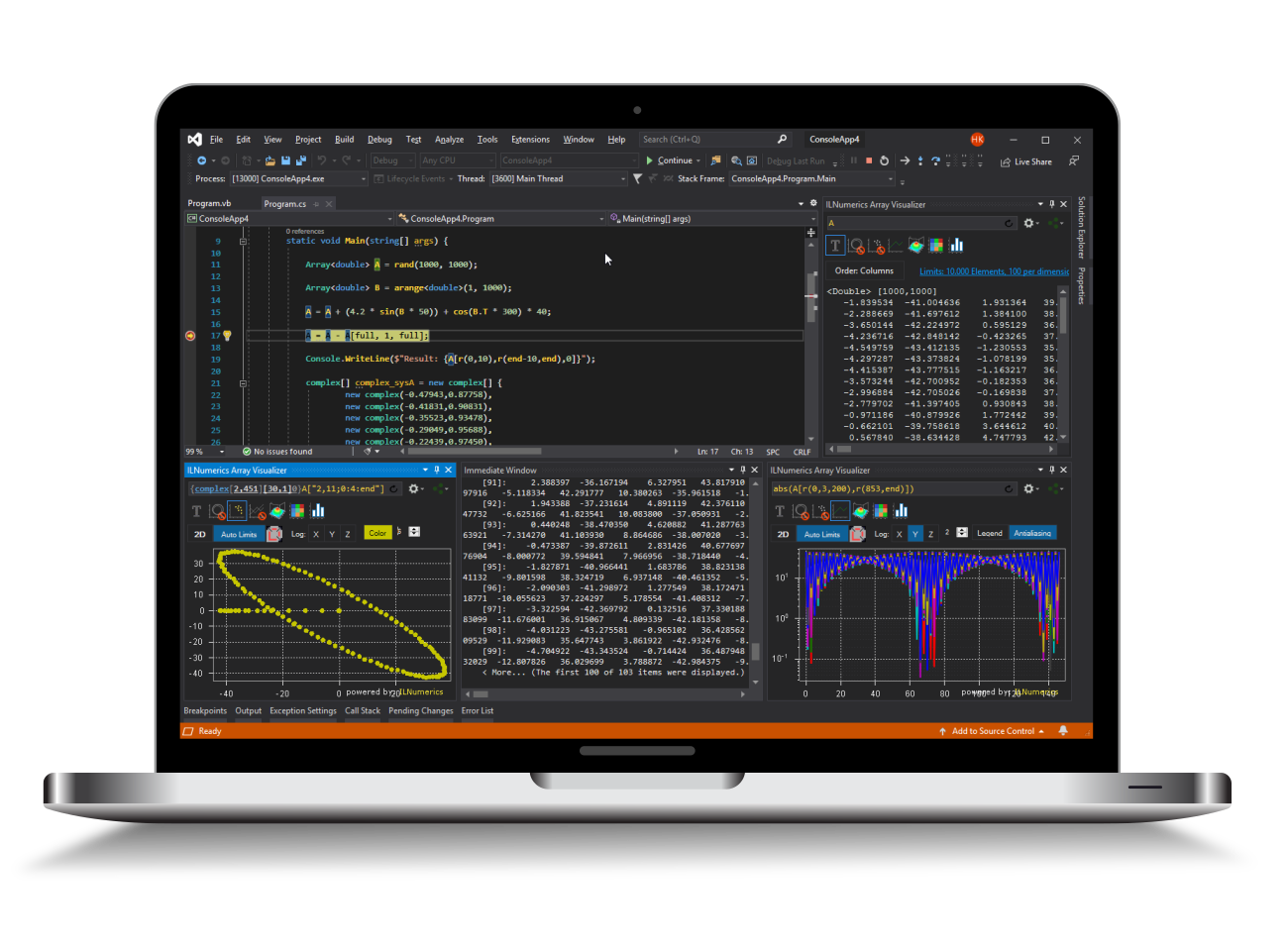Industrial Data Science
in C# and .NET:
Simple. Fast. Reliable.ILNumerics - Technical Computing

Modern High Performance Tools for Technical

Computing and Visualization in Industry and Science

tgt

## Multiple Plot Cubes

Multiple plot cubes may be used within the same panel. The PlotCube.ScreenRect property determines the rectangular area which the plot cube is occupying on the panel. This rectangle is specified in relative coordinates, ie. (0, 0, 1, 1) corresponds to the full available panel area, (0.5, 0, 0.5, 0.5) corresponds to the upper right quadrant.

Note, in order to distribute the plot cubes on the panel, internally, the ScreenRect property of the camera in every plot cube is used to create individual viewports for every plotting cube (See  Viewports for more details on how to configure individual screen rectangles.

PlotCube.ScreenRect is able to distribute plot cubes within one panel and align them according to their outer viewport dimensions. However, sometimes individual plots need to get aligned more precisely, possibly by the content rather than the outer limits. The following example of a combined plot makes this clear:

There are several problems here:

1. By altering the default space for tick labels on the Y axis the top plot decreases its data frame size. Hence, both plot cubes are not exactly aligned anymore.
2. Both plot cubes share the X axis ticks and labels. But even if their ScreenRects (Viewports) are closely arranged without any gap between them, the result appears to have a too large space between the upper and the lower plot cube. Both problems can be solved by the PlotCube.DataScreenRect property. DataScreenRect determines the rectangular area which is used by the inner data frame for a plot cube. This corresponds to the area containing the plot cube content, without any ticks and labels.

By adding 3 lines to the setup we can fix both problems:

Now, both plot cubes data frames are exactly aligned within their individual plot cube viewports.

Note that the DataScreenRect property is also given in relative units, according to the current ScreenRect of the plot cube. Configuring a height of 1 for the DataScreenRect would force the plot cube data frame to use the full available viewport height – possibly clipping ticks and labels.

## Multiple Data Groups within one Plot Cube

Plots in ILNumerics plotting cubes are organized as data groups. Each plotting cube is able to host an arbitrary number of data groups – each hosting an arbitrary number of plots.

Several properties of data groups are common for all plots contained: logarithmic/linear axis scales, the current axes limits and the axis objects associated with- and displaying those limits.

If any of these properties need to be configured individually for any two plots in the plot cube, the plots must be placed in individual data groups. New data groups are created by the PlotCube.AddDataGroup() function. By default, one data group is created for every plot cube and is accessible by the PlotCube.Plots property. Also, most members of PlotCube which affect any plots are redirected and address the first data group in the plot cube.

For example, all plots which are added to a plot cube via

are implicitly added to the first data group.

In the following example a plot cube is created which hosts two line plots. One shows some data with linear scaling. The other one shows the same data in logarithmic scaling. Both line plots are hosted within the same scene. The linear scale is used by the default data group and utilizes the default axes.

The logarithmically scaled data group uses a second Y axis on the right side of the plot cube for displaying the scale for the line plot in a red color. This line plot itself is also colored red.

For demonstration purposes, the code does use C# 2.0 syntax this time:

## Interactivity for Multiple Data Groups

When a plot cube contains more than one data group, all user interaction affects all plots within all data groups (See Plot Cube Mouse Interaction, Logarithmic Scales).